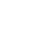# Calculation formula of color steel tile

First, the amount of color steel structure tile calculation formula

1 .side slope roof map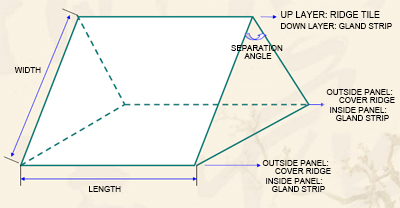Two-sided roof design
1). Roof area: length × width
2). The total length of tile: roof area ÷ 0.855 (watts effective width of 0.855M / Zhang)
3). The number of tiles: (roof length ÷ 0.855m) × 2
4). Number of ridge tile: roof length ÷ 2.4m (ridge tile effective length of 2.4M / root)
5). The number of seals: (length ÷ 0.7m) × 2 (seal 0.7M / root)
6). Number of cover covers: (length ÷ 0.7m) × 2 (cover cover 0.7M / root)
7). Number of special nails: 4PCS / ㎡
8). Roof angle ≦ 120 degrees

2. side slope roof map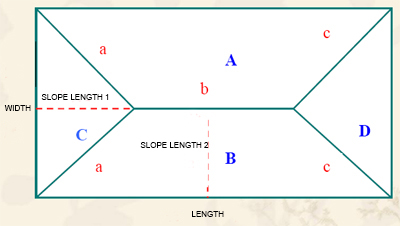Four-sided slope roof plan
1). Budget Roofing area: (A + B + C + D) x 117% (inclination and loss)
2). need tile length: roof area ÷ 0.855 (watts effective width of 0.855M / Zhang)
3). The number of ridge tile: (a × 2 + b + c × 2) ÷ 0.7 (ridge tile effective length of 2.4M / root)
4). Number of seals: (length + width) ÷ 0.7 (seal 0.7M / root)
5). Number of cover covers: (length + width) × 2 ÷ 0.7 (cover cover 0.7M / root)
6). Number of special nails: 4PCS / ㎡
7).A map area: [(b + length) × slope length 2] ÷ 2
8).B map area: with the A map area
9).C map area: (width × slope length 1) ÷ 2
10).D map area: with the C map area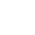v

contact us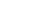DAMS Incorporated

We provide customers with quality products and provide high-quality services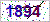If you would like to leave us a comment please go to contact us